• Hash256
• Hash160
• Reverse Bytes
• Satoshis

# ECDSA

Elliptic Curve Digital Signature Algorithm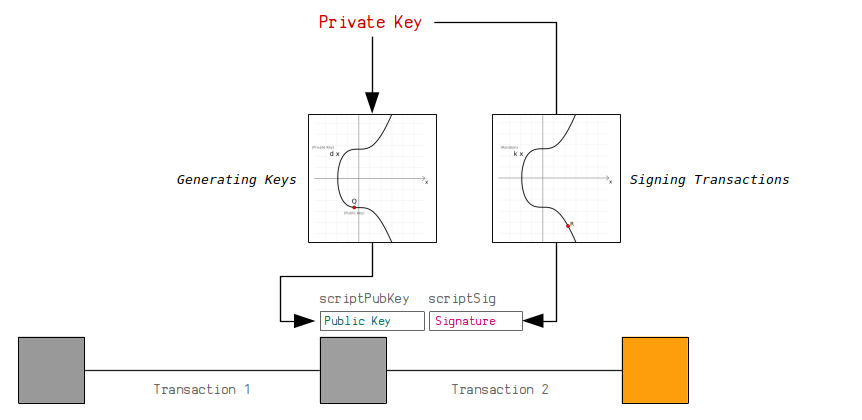Bitcoin uses a digital signature system called ECDSA to control the ownership of bitcoins.

In short, a digital signature system allows you to generate your own `private`/`public` key pair, and use the `private key` to generate `digital signatures` that proves you are the owner of the `public key` without having to reveal the `private key`.

This system is used in Bitcoin to allow people to receive and send bitcoins. Anyone can generate their own pair of keys, and then anyone can send (or “lock”) bitcoins to your `public key`. Nobody can steal these bitcoins, because only the person with the correct `private key` for this `public key` is able to generate valid signatures to “unlock” the bitcoins and send them to someone else.

The ability to create digital signatures has been around since the 1970s thanks to the invention of RSA. In 1994, DSA was released as the standard for digital signature systems. ECDSA is just an implementation of DSA using elliptic curve cryptography, as the mathematics of elliptic curves allow for more efficient signature creation and verification.

I don’t know enough about cryptography to explain why ECDSA works, but I made this page to show you how you can create your own private and public keys in Bitcoin, and use them to sign your own transactions.

The code is in Ruby, and I’ve made the diagrams and equations as simple as I can.

It wasn’t necessary for Satoshi Nakamoto to know the details of how digital signature systems work either. All they needed to know is that it does work, and that they could use it as the mechanism for sending and receiving money in the system they were building. The first version of Bitcoin used the OpenSSL library to provide the functionality for creating and verifying digital signatures.

Full ECDSA Code (Ruby)
``````require "digest" # for hashing transaction data so we can sign it
require "securerandom" # for generating random nonces when signing

# -------------------------
# Elliptic Curve Parameters
# -------------------------
# y² = x³ + ax + b
\$a = 0
\$b = 7

# prime field
\$p = 2 ** 256 - 2 ** 32 - 2 ** 9 - 2 ** 8 - 2 ** 7 - 2 ** 6 - 2 ** 4 - 1

# number of points on the curve we can hit ("order")
\$n = 115792089237316195423570985008687907852837564279074904382605163141518161494337

# generator point (the starting point on the curve used for all calculations)
\$G = {
x: 55066263022277343669578718895168534326250603453777594175500187360389116729240,
y: 32670510020758816978083085130507043184471273380659243275938904335757337482424,
}

# ---------------
# Modular Inverse: Ruby doesn't have a built-in modinv function
# ---------------
def inverse(a, m = \$p)
m_orig = m         # store original modulus
a = a % m if a < 0 # make sure a is positive
prevy, y = 0, 1
while a > 1
q = m / a
y, prevy = prevy - q * y, y
a, m = m % a, a
end
return y % m_orig
end

# ------
# Double: add a point to itself
# ------
def double(point)
# slope = (3x₁² + a) / 2y₁
slope = ((3 * point[:x] ** 2 + \$a) * inverse((2 * point[:y]), \$p)) % \$p # using inverse to help with division

# x = slope² - 2x₁
x = (slope ** 2 - (2 * point[:x])) % \$p

# y = slope * (x₁ - x) - y₁
y = (slope * (point[:x] - x) - point[:y]) % \$p

# Return the new point
return { x: x, y: y }
end

# ---
# ---
# double if both points are the same
if point1 == point2
return double(point1)
end

# slope = (y₁ - y₂) / (x₁ - x₂)
slope = ((point1[:y] - point2[:y]) * inverse(point1[:x] - point2[:x], \$p)) % \$p

# x = slope² - x₁ - x₂
x = (slope ** 2 - point1[:x] - point2[:x]) % \$p

# y = slope * (x₁ - x) - y₁
y = ((slope * (point1[:x] - x)) - point1[:y]) % \$p

# Return the new point
return { x: x, y: y }
end

# --------
# Multiply: use double and add operations to quickly multiply a point by an integer value (i.e. a private key)
# --------
def multiply(k, point = \$G)
# create a copy the initial starting point (for use in addition later on)
current = point

# convert integer to binary representation
binary = k.to_s(2)

# double and add algorithm for fast multiplication
binary.split("").drop(1).each do |char| # from left to right, ignoring first binary character
# 0 = double
current = double(current)

# 1 = double and add
current = add(current, point) if char == "1"
end

# return the final point
current
end

# ----
# Sign
# ----
def sign(private_key, hash, nonce = nil)
# generate random number if not given
if nonce == nil
loop do
nonce = SecureRandom.hex(32).to_i(16)
break if nonce < \$n # make sure random numer is below the number of points of the curve
end
end

# r = the x value of a random point on the curve
r = multiply(nonce)[:x] % \$n

# s = nonce⁻¹ * (hash + private_key * r) mod n
s = (inverse(nonce, \$n) * (hash + private_key * r)) % \$n # this breaks linearity (compared to schnorr)

# signature is [r, s]
return { r: r, s: s }
end

# ------
# Verify
# ------
def verify(public_key, signature, hash)
# point 1
point1 = multiply(inverse(signature[:s], \$n) * hash)

# point 2
point2 = multiply((inverse(signature[:s], \$n) * signature[:r]), public_key)

# add two points together
point3 = add(point1, point2)

# check x coordinate of this point matches the x-coordinate of the random point given
return point3[:x] == signature[:r]
end

# -------------------
# Create A Public Key
# -------------------
# Example private key (in hexadecimal)
private_key = "f94a840f1e1a901843a75dd07ffcc5c84478dc4f987797474c9393ac53ab55e6"

# Public key is the generator point multiplied by the private key
point = multiply(private_key.to_i(16))

# convert x and y values of the public key point to hexadecimal
x = point[:x].to_s(16).rjust(64, "0") # pad with zeros to make sure it's 64 characters (32 bytes)
y = point[:y].to_s(16).rjust(64, "0")

# uncompressed public key (use full x and y coordinates) OLD FORMAT!
public_key_uncompressed = "04" + x + y

# compressed public key (use a prefix to indicate whether y is even or odd)
if (point[:y] % 2 == 0)
public_key_compressed = "02" + x # y is even
else
public_key_compressed = "03" + x # y is odd
end

#puts public_key_compressed #=> 024aeaf55040fa16de37303d13ca1dde85f4ca9baa36e2963a27a1c0c1165fe2b1

# ------------------
# Sign A Transaction
# ------------------
# A basic structure for holding the transaction data
def tx(scriptsig)
# Need to calculate a byte indicating the size of upcoming scriptsig in bytes (rough code but does the job)
size = (scriptsig.length / 2).to_s(16).rjust(2, "0")

# Raw unsigned transaction data with the scriptsig field (you need to know the correct position)
return "0100000001b7994a0db2f373a29227e1d90da883c6ce1cb0dd2d6812e4558041ebbbcfa54b00000000#{size}#{scriptsig}ffffffff01983a0000000000001976a914b3e2819b6262e0b1f19fc7229d75677f347c91ac88ac00000000"
end

# Private key and public key for the locked up bitcoins we want to spend
private_key = "f94a840f1e1a901843a75dd07ffcc5c84478dc4f987797474c9393ac53ab55e6" # sha256("learnmeabitcoin1")
public_key = "024aeaf55040fa16de37303d13ca1dde85f4ca9baa36e2963a27a1c0c1165fe2b1"

# NOTE: Need to remove all existing signatures from the transaction data first if there are any

# Put original scriptpubkey as a placeholder in to the scriptsig for the input you want to sign (required)
scriptpubkey = "76a9144299ff317fcd12ef19047df66d72454691797bfc88ac" # just one input in this transaction
transaction = tx(scriptpubkey)

# Append sighash type to transaction data (required)
transaction = transaction + "01000000"

# Get a hash of the transaction data (because we sign the hash of data and not the actual data itself)
hash = Digest::SHA256.hexdigest(Digest::SHA256.digest([transaction].pack("H*")))

# Use elliptic curve mathematics to sign the hash using the private key and nonce
signature = sign(private_key.to_i(16), hash.to_i(16), 123456789) # using a fixed nonce for testing (unsafe)

# Use the low s value (BIP 62: Dealing with malleability)
if (signature[:s] > \$n / 2)
signature[:s] = \$n - signature[:s]
end

# Encode the signature in to DER format (slightly awkward format used for signatures in bitcoin transactions)
r = signature[:r].to_s(16).rjust(64, "0")  # convert r to hexadecimal
s = signature[:s].to_s(16).rjust(64, "0")  # convert s to hexadecimal
r = "00" + r if (r[0, 2].to_i(16) >= 0x80) # prepend 00 if first byte is 0x80 or above (DER quirk)
s = "00" + r if (s[0, 2].to_i(16) >= 0x80) # prepend 00 if first byte is 0x80 or above (DER quirk)
der = ""                                   # string for holding our der encoding
r_len = (r.length / 2).to_s(16).rjust(2, "0") # get length of r (in bytes)

s_len = (s.length / 2).to_s(16).rjust(2, "0") # get length of s (in bytes)
der << "02" << r_len << r << "02" << s_len << s   # Add to DER encoding (0x20 byte indicates an integer type in DER)
der_len = (der.length / 2).to_s(16).rjust(2, "0") # get length of DER data (in bytes)
der = "30" + der_len + der # Final DER encoding (0x30 byte incatetes compound object type)

# Append sighashtype to the signature (required) (01 = ALL)
der = der + "01" # without it you get "mandatory-script-verify-flag-failed (Non-canonical DER signature) (code 16)"

# Contruct full unlocking script (P2PKH scripts need original public key the bitcoins were locked to): <size> {signature} <size> {public_key}
scriptsig = (der.length / 2).to_s(16) + der + (public_key.length / 2).to_s(16) + public_key

# Put the full scriptsig in to the original transaction data
transaction = tx(scriptsig)

# Show the signed transaction
puts transaction #=> 0100000001b7994a0db2f373a29227e1d90da883c6ce1cb0dd2d6812e4558041ebbbcfa54b000000006a473044022008f4f37e2d8f74e18c1b8fde2374d5f28402fb8ab7fd1cc5b786aa40851a70cb02201f40afd1627798ee8529095ca4b205498032315240ac322c9d8ff0f205a93a580121024aeaf55040fa16de37303d13ca1dde85f4ca9baa36e2963a27a1c0c1165fe2b1ffffffff01983a0000000000001976a914b3e2819b6262e0b1f19fc7229d75677f347c91ac88ac00000000

# Send the transaction in to the bitcoin network
# \$ bitcoin-cli sendrawtransaction [raw transaction data]``````

## Elliptic Curves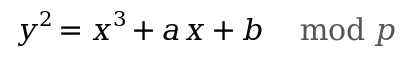ECDSA uses an elliptic curve as the basis for a digital signature system.

In summary, `public keys` and `signatures` are just points on an elliptic curve. If both of these points are created from the same `private key` (a large number), there will be a geometric connection between them that proves that the person who created the `signature` also created (or “owns”) the `public key` too.

### Parameters

There are a number of different curves that can be used in ECDSA. Satoshi chose the secp256k1 curve, which has the following parameters:

``````# y² = x³ + ax + b
\$a = 0
\$b = 7

# prime field
\$p = 2 ** 256 - 2 ** 32 - 2 ** 9 - 2 ** 8 - 2 ** 7 - 2 ** 6 - 2 ** 4 - 1

# number of points on the curve we can hit ("order")
\$n = 115792089237316195423570985008687907852837564279074904382605163141518161494337

# generator point (the starting point on the curve used for all calculations)
\$G = {
x: 55066263022277343669578718895168534326250603453777594175500187360389116729240,
y: 32670510020758816978083085130507043184471273380659243275938904335757337482424,
}``````
• An elliptic curve is a set of points described by the equation `y² = x³ + ax + b`, so this is where the `a` and `b` variables come from. Different curves will have different values for these coefficients, and `a=0` and `b=7` are the ones specific to secp256k1.
• The prime modulus `p` is just a number that keeps all of the numbers within a specific range when performing mathematical calculations (again it’s specific to secp256k1). The fact that it’s a prime number is a key ingredient for the cryptography to work, but that’s an aside.
• There are `n` number of points on the curve we can reach. This is also referred to as the “order”. It’s less than `p`, and it’s based on the chosen generator point (see below).
• Finally, every curve has a generator point `G`, which is basically the starting point on the curve used when performing most mathematical operations. The exact origin for the choice of this point is unknown1, but it’s usually because it provides a high order (see above) and has shown to not have any inherent cryptographic weaknesses.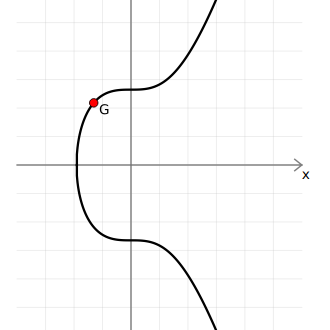Every curve has a generator point G.
Note: Elliptic curves over a finite field.

The diagrams I’m using in this tutorial show a smooth elliptic curve like this: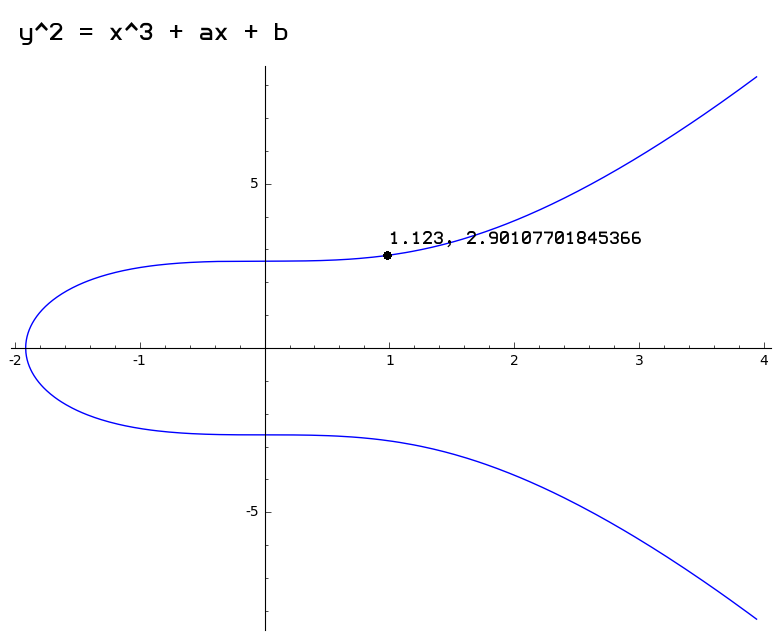Elliptic curve over real numbers (showing example point at x=1.123, y=2.90107701845366)

However, the actual curve used in Bitcoin looks more like a scatter plot of points like this: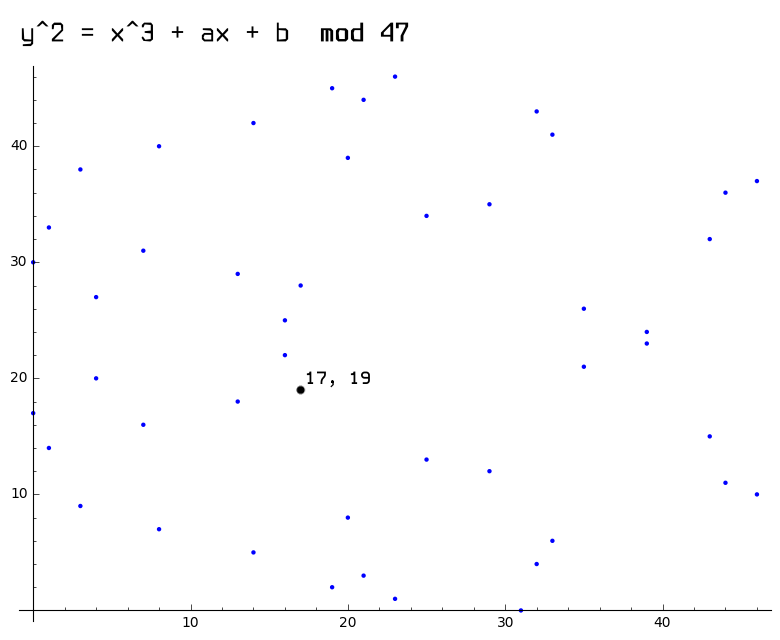Elliptic curve over a finite field mod 47 (showing example point at x=17, y=19)

This is due to the fact that the curve used in Bitcoin is over a finite field of whole numbers (i.e. using `mod p` to restrict numbers to within a certain range), and this breaks the continuous curve you’re able to get when you use real numbers. However, even though these plots look wildly different, the mathematical operations you can perform on both of these curves will still work in the same way.

Of course, the secp256k1 curve has a very large value for `p`, so it more closely resembles a graph that looks like the following, except imagine there are about as many points on it as there are atoms in the universe: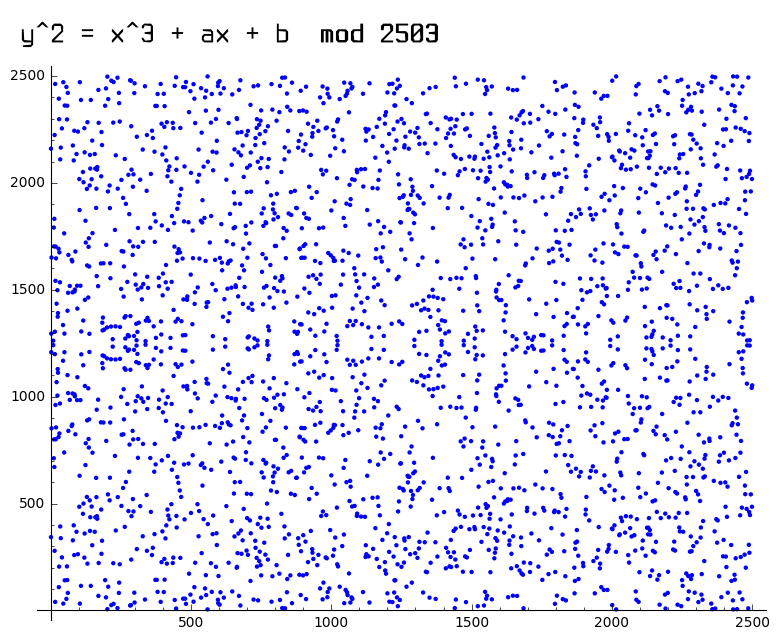Elliptic curve over a finite field mod 2503

But why use finite fields?

Because, when implementing cryptography on computers, it’s easier to work with the whole numbers in a finite field (e.g. `1, 2, 3, 4, ..., p`), than it is to work with an infinite amount of the real numbers (e.g. `0.911722707844879, 2.90107701845366, ...`). You risk losing accuracy when working with all these decimal numbers on a computer, so the precision you get with a finite set of whole numbers is better suited for cryptography.

For the rest of this tutorial I’ll be showing the smooth curve because it looks better for illustrative purposes.
Note: Why is it called secp256k1?

This is a nickname for one of the specific curves used in elliptic curve cryptography:

• sec = Standard for Efficient Cryptography — A consortium that develops commercial standards for cryptography.
• p = Prime — The prime number used to create the finite field.
• 256 = 256 bits — Size of the prime field used.
• k = Koblitz — Specific type of curve.
• 1 = First curve in this category.

## Elliptic Curve Mathematics

There are a few mathematical operations that you can perform on points on the elliptic curve. The main two operations are `double()` and `add()`, and these can then be combined to perform `multiply()`.

These operations are the building blocks of elliptic curve cryptography, and they are used for generating `public keys` and `signatures` in ECDSA.

### Modular Inverse

Before being able to perform `double()` and `add()` operations on points on the curve, we first need to be able to find the modular inverse of a number in a finite field (bare with me).

You see, there is no actual straightforward “division” operation in elliptic curve cryptography because all of the mathematics takes place within a of numbers.

However, in a finite field you can multiply by the inverse of a number to achieve the same result as division.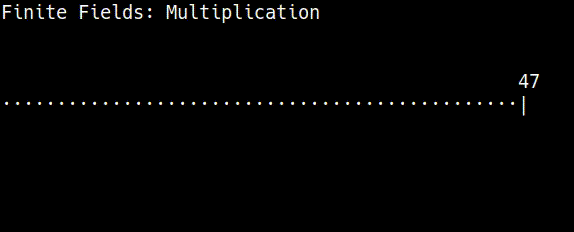29 is the modular multiplicative inverse of 13 in the finite field of 47.

In other words, if you start at a specific number in a finite field and multiply by another number, you can “go backwards” to the number you started with by multiplying again by the inverse of the number you used for multiplication.

This always works when you have a prime number of elements in the field. A prime number cannot be divided by any other number, so it will distribute the results from modular multiplication back across each of the numbers in the field evenly (without repeating and missing some numbers). So by using a prime number as the modulus you can guarantee that each number in the finite field will have a multiplicative inverse (or a “division” operation).

Obviously this is a confusing first step in to elliptic curve math, but just think of “finding the inverse” as a basic tool in modular arithmetic.

Not all programming languages have a built-in “modular inverse” function though, which is why you sometimes have to implement one yourself to get started with elliptic curve mathematics, such as in Ruby:

``````def inverse(a, m = \$p)
m_orig = m         # store original modulus
a = a % m if a < 0 # make sure a is positive
prevy, y = 0, 1
while a > 1
q = m / a
y, prevy = prevy - q * y, y
a, m = m % a, a
end
return y % m_orig
end``````

This function uses the extended Euclidean algorithm (you don’t need to know how it works) to find the modular inverse of a number. It’s just a quicker method than trying to find the inverse via brute-force.

The upcoming `double()` and `add()` equations include division `/`, which is why we need to be able to work out the modular inverse of a number (in a prime field) before moving forward.

The modular inverse of a number is typically denoted by `⁻¹` in mathematical equations.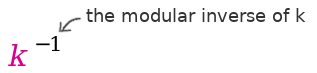In the upcoming mathematics, the inverse of a number is sometimes found `mod p` (modulo the prime), and is sometimes found `mod n` (modulo the number of points on the curve). It depends on which modulus is being used for the equation.

### Double

“Doubling” a point is the same thing as “adding” a point to itself.

From a visual perspective, to “double” a point you draw a tangent to the curve at the given point, then find the point on the curve this line intersects (there will only be one), then take the reflection of this point across the x-axis.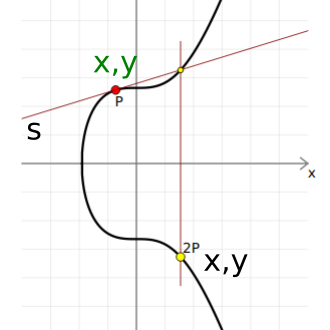P is just the letter used for a general point on the curve.s here refers to the slope of the tangent.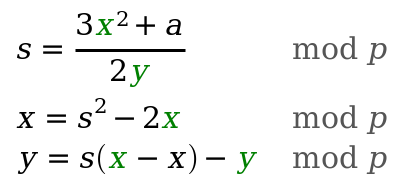Elliptic curve point doubling.

Elliptic Curve Operations.

As you can see, you’re not actually “doubling” the values of the `x, y` coordinates of a point here (like you would do in everyday arithmetic). The “double”, “add”, and “multiply” terms on this page refer to specific operations we perform with points on elliptic curves. So even though they have the same names as normal mathematical operations, understand that they are completely different when in the domain of elliptic curve mathematics.

This can get a bit confusing at times because there are also everyday “add” and “multiply” operations in amongst these equations too. The trick is to remember that every time one of these operations is on a point, we’re using the elliptic curve operations. And when these operations are on two integers, it’s just everyday arithmetic.

``````def double(point)
# slope = (3x₁² + a) / 2y₁
slope = ((3 * point[:x] ** 2 + \$a) * inverse((2 * point[:y]), \$p)) % \$p # using inverse to help with division

# x = slope² - 2x₁
x = (slope ** 2 - (2 * point[:x])) % \$p

# y = slope * (x₁ - x) - y₁
y = (slope * (point[:x] - x) - point[:y]) % \$p

# Return the new point
return { x: x, y: y }
end``````

As expected, “addition” of two points in elliptic curve mathematics isn’t the same as straightforward integer addition, but it’s called “addition” anyway.

From a visual perspective, to “add” two points together you draw a line between them, then find the point on the curve this line intersects (there will only be one), then take the reflection of this point across the x-axis.

``````def add(point1, point2)
# double if both points are the same
if point1 == point2
return double(point1)
end

# slope = (y₁ - y₂) / (x₁ - x₂)
slope = ((point1[:y] - point2[:y]) * inverse(point1[:x] - point2[:x], \$p)) % \$p

# x = slope² - x₁ - x₂
x = (slope ** 2 - point1[:x] - point2[:x]) % \$p

# y = slope * (x₁ - x) - y₁
y = ((slope * (point1[:x] - x)) - point1[:y]) % \$p

# Return the new point
return { x: x, y: y }
end``````

### Multiply

Now that we can “double” and “add” points on the curve, we can now take any point on the curve and “multiply” it by an integer to get to a completely new point. This operation is the heart of elliptic curve cryptography.

The simplest method for elliptic curve multiplication would be to repeatedly “add” a point to itself until you reach the number you want to multiply by, which would work to a degree, but these incremental `add()` operations would make this approach impossibly slow when multiplying by large numbers (like the ones used in Bitcoin).

Thankfully there is a faster way to perform multiplication on elliptic curves…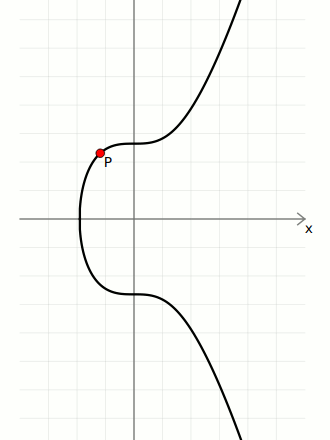3 * P is the same as one double() operation and one add() operation: 3P = 2P + P

A faster approach to multiplication is to use the double-and-add algorithm, where you make an efficient use of both doubling and adding to reach the target multiple in as few operations as possible.

For example, if you start at `2` and want to get to `128`, it’s faster to perfom six `double()` operations than it is to perform sixty-four `add()` operations.

But how do you know how many double and add operations you need to get to your target multiple?

Well, amazingly, if you convert any integer in to its binary representation, the `1`s and `0`s will provide a map for the sequence of `double()` and `add()` operations you need to perform to reach that multiple.

Working from left to right and ignoring the first number:

• `0` = double
• `1` = double and add

For example:

``````e.g. 1 * 21

21 = 10101 (binary)
│││└ double and add = 21
││└─ double         = 10
│└── double and add = 5
└─── double         = 2
1  <- start here``````

Anyway, here’s what elliptic curve multiplication looks like when using the double-and-add algorithm in Ruby code:

``````def multiply(k, point = \$G)
# create a copy the initial starting point (for use in addition later on)
current = point

# convert integer to binary representation
binary = k.to_s(2)

# double and add algorithm for fast multiplication
binary.split("").drop(1).each do |char| # from left to right, ignoring first binary character
# 0 = double
current = double(current)

# 1 = double and add
current = add(current, point) if char == "1"
end

# return the final point
current
end``````

Most multiplication operations in ECDSA start at the generator point `G`.

Every multiplication operation starts with a `double()`. This is because you’re starting with a single point (e.g. the generator point).

## ECDSA

Now that we know about the structure of elliptic curves and we have a `multiply()` operation for points, we can actually use this as the basis for a system for creating digital signatures.

The following system is called the Elliptic Curve Digital Signature Algorithm, or ECDSA for short.

### Key Generation

We create pairs of keys using elliptic curve multiplication:

• `private key` `d` — A large randomly-generated number between `[0...n-1]`
• `public key` `Q` — The generator point `G` multiplied by this random number `d`.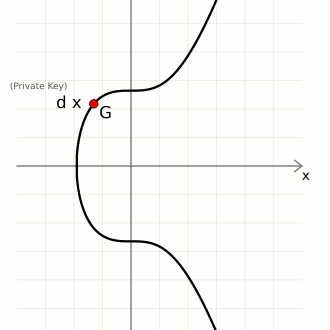d is the private key (an integer)G is the generator point (a point)Q is the public key (a point)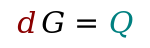So in elliptic curve cryptography, a `private key` is just a large random integer (less than the number of points on the curve), and its corresponding `public key` is just a point on the curve.

For example:

``````private key = 112757557418114203588093402336452206775565751179231977388358956335153294300646
public key  = {
x: 33886286099813419182054595252042348742146950914608322024530631065951421850289,
y: 9529752953487881233694078263953407116222499632359298014255097182349749987176
}
``````
Trapdoor Function: You can give your `public key` to anyone, and your `private key` will remain secret.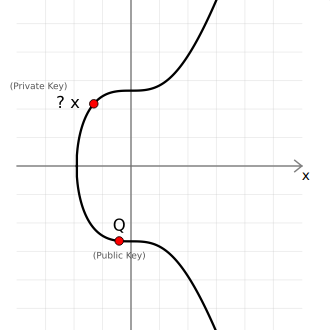An important point (heh) to note here is that given a `public key` point `Q`, there’s no easy way to work out the `private key` `d` used to create it. The only way to work out the `private key` would be to manually multiply the generator point `G` by different numbers to see if you can get the same public key, and this brute-force approach is going to be impossibly slow if someone has used a very large number for their private key.

Therefore elliptic curve multiplication is known as a trapdoor function (because it’s easy to go one way but difficult to go the other), which is a key component of all public key cryptography.

Furthermore, the one-way mathematical connection between the `private key` and `public key` means that you can use both independently to calculate the same points on the elliptic curve later on, which comes in very handy when constructing a system for creating digital signatures.

### Sign

To sign a message you need three things:

1. Random Number `k` — This introduces an element of randomness in to our signatures, which is important for security. It means that every signature we generate will be different, even if we sign the same message twice.
2. Message Hash `z` — This is the hash of the message we want to sign. Hashing the message gives us a small and unique fingerprint for it, and it’s more efficient to sign this fingerprint than it is to sign a large blob of data. You have a choice of which hash algorithm to use, but the one most commonly used with secp256k1 is `SHA-256`.
3. `Private Key` `d` — The source of a `public key` (that we’ve made publicly available).

An actual `signature` is then made of two parts:

• `r`A random point on the curve. We take the random number `k` and multiply it by the generator point to get a random point `R`. We only actually use the x-coordinate of this point, and we call this lowercase `r`.
• `s`A number to accompany the random point. This is a unique number created from a combination of the message hash `z` and `private key` `d`, which is also bound to the random point using `r`.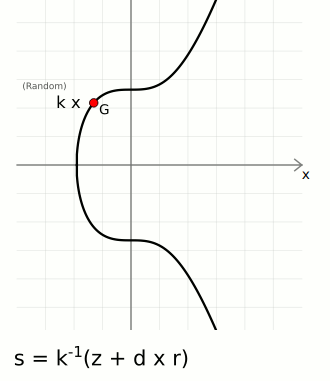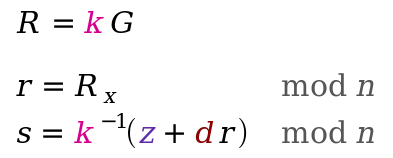The `⁻¹` notation indicates the modular inverse of that number. Here the modular multipicative inverse is found `mod n` (the number of points on the curve).

These two `[r, s]` values are the “digital signature”.

For example:

``````random number   (k): 12345
message:             ECDSA is the most fun I have ever experienced
sha256(message) (z): 103318048148376957923607078689899464500752411597387986125144636642406244063093
private key     (d): 112757557418114203588093402336452206775565751179231977388358956335153294300646

random point (k*G = R): {
x = 108607064596551879580190606910245687803607295064141551927605737287325610911759,
y = 6661302038839728943522144359728938428925407345457796456954441906546235843221
}
signature: r = R[x], s = k⁻¹ * (z + r * d): {
r = 108607064596551879580190606910245687803607295064141551927605737287325610911759,
s = 73791001770378044883749956175832052998232581925633570497458784569540878807131
}
``````

In short, the unique `s` value provides a pathway for getting to the randomly-generated point `r`.

You can give these two pieces of information to someone else, and starting from the `public key` point `Q` they can use the `s` value to help them get to the random point `r`. The trick here is that only the person with the corresponding `private key` `d` could create a valid pathway to this random point provided by `s`.

This pathway also has the message hash `z` encoded in to it, which is what effectively allows us to create signatures for messages; nobody can create the pathways from the `public key` to a random point on the curve via the message hash without knowing the `private key` it was created from.

``````def sign(private_key, hash, nonce = nil)
# generate random number if not given
if nonce == nil
loop do
nonce = SecureRandom.hex(32).to_i(16)
break if nonce < \$n # make sure random numer is below the number of points of the curve
end
end

# r = the x value of a random point on the curve
r = multiply(nonce)[:x] % \$n

# s = nonce⁻¹ * (hash + private_key * r) mod n
s = (inverse(nonce, \$n) * (hash + private_key * r)) % \$n # this breaks linearity (compared to schnorr)

# signature is [r, s]
return { r: r, s: s }
end``````

Nonce: A random number in cryptography is sometimes called a “nonce”, which stands for “number used once”.

Why do you need to generate a random point each time? (Mathematical Explanation)

If you use the same value for `k` more than once (i.e. you use the same random point for more than one signature), anyone can actually work out your `private key` from your signatures.

For example, lets say we’re given two signed messages that were generated using the same value for `k`.

For each signed message we have the message hash `z`, and also the `r` and `s` values from each of the respective signatures:

``````Signed Message 1: (z₁, r₁, s₁)
Signed Message 2: (z₂, r₂, s₂)``````

However, because the same value for `k` was used each time to generate the random point (`R = k*G`), the `r` value (x-coordinate of `R`) in each of these signatures will also be the same:

``````Signed Message 1: (z₁, r, s₁)
Signed Message 2: (z₂, r, s₂)``````

So how can we use this information to work out the private key `d`?

First of all, we know that the `s` value in each of these signatures was calculated using `s = k⁻¹(z + r * d) mod n`, so:

``````s₁ = k⁻¹(z₁ + r * d) mod n
s₂ = k⁻¹(z₂ + r * d) mod n``````

And thanks to the fact that both equations now have the same value for `k`, we can solve them as a pair of simultaneous equations to work out the value for `k`.

To do this, we start by rearranging the second equation to get `r * d` on its own:

``````s₂ = k⁻¹(z₂ + r * d) mod n
r * d = k * s₂ - z₂ mod n``````

Then we can substitute this in to the first equation, and rearrange it to get `k`:

``````s₁ = k⁻¹(z₁ + r * d) mod n
s₁ = k⁻¹(z₁ + (k * s₂ - z₂)) mod n
k = (z₁ - z₂) * (s₁ - s₂)⁻¹ mod n``````

Remember that multiplying by `(s₁ - s₂)⁻¹` means multiplying by the modular multiplicative inverse of `(s₁ - s₂)`, which is the same thing as “division” in elliptic curve mathematics.

And after we’ve worked out `k`, we can use it in `s = k⁻¹(z + r * d) mod n` again to work out `d`.

So rearranging the first equation (you can use either) to get `d` on its own:

``````s₁ = k⁻¹(z₁ + r * d) mod n
d = (k * s₁ - z₁) * r⁻¹ mod n``````

And because we already knew `(z₁, r, s₁)` and have just worked out `k`, we can plug them all in to this equation to work out the private key `d`.

In mathematical notation, the private key recovery looks like this: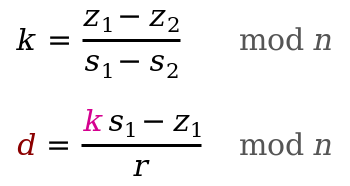So make sure you always use securely random values for `k` each time you create a signature. If someone spots you’ve used the same `r` value when signing different messages for the same public key, it only takes milliseconds for them to recover your private key.

In 2011 hackers worked out how to get the private key for the PS3 because Sony were using the same value for `k` when generating their signatures.

Here’s an example of recovering a private key from two signatures using the same `k` in Ruby:

``````require 'digest' # used for hashing messages before signing

# Note: This code uses the previously defined inverse(), double(), add(), multiply(), and sign() functions

# -------------
# Sign Messages
# -------------

# 0. Keys
prv = 1111222233334444555566667777888899990000 # any old private key
pub = multiply(prv)

# 1. Create first signed message
k    = 800000
z1   = Digest::SHA256.hexdigest("Just a simple message.").to_i(16)
sig1 = sign(prv, z1, k)

# 2. Create second signed message
k    = 800000 # Using the same value for k!
z2   = Digest::SHA256.hexdigest("I have used the same k value.").to_i(16)
sig2 = sign(prv, z2, k)

# --------------------
# Private Key Recovery
# --------------------
# k = (z₁ - z₂) * (s1 - s₂)⁻¹  mod n
# d = (k * s₁ - z₁) * r⁻¹    mod n

# 1. Work out k (note: result may be the additive inverse of original k, but it still works fine)
k_calculated = ((z1 - z2) * inverse(sig1[:s] - sig2[:s], \$n)) % \$n

# 2. Work out d (the original private key)
d_calculated = ((k_calculated * sig1[:s] - z1) * inverse(sig1[:r], \$n)) % \$n
puts d_calculated #=> 1111222233334444555566667777888899990000``````

### Verify

You can verify a message and its signature with three things:

1. `Public Key` `Q` — This is the public key for the person claiming to have created the signature.
2. Message — The data that was signed. We can hash it ourselves to get the message hash `z`.
3. Signature `[r, s]` — This is the signature created for the above message, allegedly created by the person who has the `private key` for the `public key`.

We then use these three pieces of data to calculate two points on the curve:

• Point 1. Start with the generator point `G`, and multiply it by `inverse(s) * z`.
• Point 2. Start with the `public key` point `Q`, and multiply it by `inverse(s) * r`.

We can now add these points together to give us Point 3: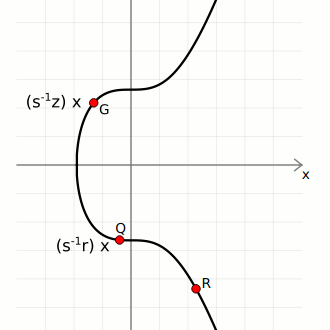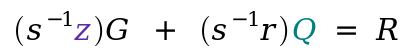If this third point matches up with the random point given, the signature is valid.

For example:

``````message:             ECDSA is the most fun I have ever experienced
sha256(message) (z): 103318048148376957923607078689899464500752411597387986125144636642406244063093
signature (r,s): {
r = 108607064596551879580190606910245687803607295064141551927605737287325610911759,
s = 73791001770378044883749956175832052998232581925633570497458784569540878807131
}
public key (Q): {
x = 33886286099813419182054595252042348742146950914608322024530631065951421850289,
y = 9529752953487881233694078263953407116222499632359298014255097182349749987176
}

verification (s⁻¹ * z)G + (s⁻¹ * r)Q: {
x = 108607064596551879580190606910245687803607295064141551927605737287325610911759, <- matches r (x-coordinate of random point)
y = 6661302038839728943522144359728938428925407345457796456954441906546235843221
}``````

In other words, the signature for this message could only have been created by the person who has the actual `private key` that the `public key` was created from. Nobody else can give you an `s` value that you can use in combination with the `public key` `Q` to reach the random point `R` unless they knew the `private key` `d` for that public key.

If you change the contents of the signed message or try to use the signature with a different `public key`, the resulting third point won’t match up with the random point given in the signature, and the signature verification will fail.

``````def verify(public_key, signature, hash)
# point 1
point1 = multiply(inverse(signature[:s], \$n) * hash)

# point 2
point2 = multiply((inverse(signature[:s], \$n) * signature[:r]), public_key)

# add two points together
point3 = add(point1, point2)

# check x coordinate of this point matches the x-coordinate of the random point given
return point3[:x] == signature[:r]
end``````
Why does this work? (Mathematical Explanation)

Signing:

The person creating a signature starts by using a random number `k` to generate a random point on the curve:

``R = k * G``

They then compute an auxillary number using their private key `d` and the hash of the message `z` (along with `r` (the x-coordinate of `R`) and the random number `k`):

``s = k⁻¹ * (z + r * d)``

Verifying:

The following equation allows you to calculate the same point by using the public key `Q` alongside the hash of the message `z` and the given `s` value:

``R = (s⁻¹ * z)G + (s⁻¹ * r)Q``

We can now rearrange this equation and substitute some values to prove that this equation does indeed get us to the same point.

To start with, the public key `Q` is `d * G`, so:

``R = (s⁻¹ * z)G + (s⁻¹ * r)d*G``

If we rearrange this equation we get:

``````R = (s⁻¹ * z)G + (s⁻¹ * r * d)G
R = s⁻¹ * (z + r * d) * G``````

Now, remember that `s = k⁻¹ * (z + r * d)`. If we rearrange this to get `k` on its own we get `k = s⁻¹ * (z + r * d)`, and substituting this in to the equation above:

``R = k * G``
And that’s the same calculation that was used to generate the random point in the first place.

## ECDSA in Bitcoin

ECDSA is used in Bitcoin when:

In both of these situations you’re just using the exact same elliptic curve mathematics as above.

Most of the time the only tricky part is getting the data in to the correct format.

### 1. Creating a public keyA public key can be put inside the locking script on top of an output.

A `public key` is just the generator point multiplied by your `private key`.

So it’s a point on the curve made up of `x` and `y` coordinates:

``````private key = 112757557418114203588093402336452206775565751179231977388358956335153294300646
public key  = {
x: 33886286099813419182054595252042348742146950914608322024530631065951421850289,
y: 9529752953487881233694078263953407116222499632359298014255097182349749987176
}
``````

When converting this point in to the common format used in Bitcoin, you essentially just convert each coordinate to hexadecimal:

``````public key  = {
x: 4aeaf55040fa16de37303d13ca1dde85f4ca9baa36e2963a27a1c0c1165fe2b1,
y: 1511a626b232de4ed05b204bd9eccaf1b79f5752e14dd1e847aa2f4db6a52768
}

public key = 4aeaf55040fa16de37303d13ca1dde85f4ca9baa36e2963a27a1c0c1165fe2b11511a626b232de4ed05b204bd9eccaf1b79f5752e14dd1e847aa2f4db6a52768
``````

Don’t forget to pad each hexadecimal coordinate to 32 bytes (64 characters) by prepending zeros if necessary.

However, one interesting thing about the elliptic curve used in ECDSA is that every `x` coordinate will have one of two possible `y` coordinates (one is even and the other is odd).

So instead of having to use the full `y` coordinate all the time, we can use a prefix to indicate which of the two possible `y` coordinates we’re using for a given point. This halves the size of a formatted public key, and reduces the amount of data required when building transactions.

``````Public key prefixes:

02 = compressed (y is even)
03 = compressed (y is odd)
04 = uncompressed (full y is included)
``````

With our example public key we can see that the `y` coordinate is even, so we can just use a `02` prefix alongside the full `x` coordinate to shorten the encoded public key:

``````public key (uncompressed) = 044aeaf55040fa16de37303d13ca1dde85f4ca9baa36e2963a27a1c0c1165fe2b11511a626b232de4ed05b204bd9eccaf1b79f5752e14dd1e847aa2f4db6a52768
public key (compressed)   = 024aeaf55040fa16de37303d13ca1dde85f4ca9baa36e2963a27a1c0c1165fe2b1
``````

These shortened public keys are called compressed public keys, and they’re the preferred format for use in Bitcoin.

And finally, as mentioned, these public keys can then be used for receiving bitcoin by putting them inside a scriptPubKey (the “locking script”) on an output in a transaction. This effectively encumbers a fixed amount of bitcoin with a public key, and only the owner of this public key will be able to spend them later on.

The following code shows how you can convert a `private key` in to a compressed `public key` that can be used for receiving bitcoin:

``````# Example private key (in hexadecimal)
private_key = "f94a840f1e1a901843a75dd07ffcc5c84478dc4f987797474c9393ac53ab55e6"

# Public key is the generator point multiplied by the private key
point = multiply(private_key.to_i(16))

# convert x and y values of the public key point to hexadecimal
x = point[:x].to_s(16).rjust(64, "0") # pad with zeros to make sure it's 64 characters (32 bytes)
y = point[:y].to_s(16).rjust(64, "0")

# uncompressed public key (use full x and y coordinates) OLD FORMAT!
public_key_uncompressed = "04" + x + y

# compressed public key (use a prefix to indicate whether y is even or odd)
if (point[:y] % 2 == 0)
public_key_compressed = "02" + x # y is even
else
public_key_compressed = "03" + x # y is odd
end

#puts public_key_compressed #=> 024aeaf55040fa16de37303d13ca1dde85f4ca9baa36e2963a27a1c0c1165fe2b1``````

A `private key` is just a large randomly generated number. If you’ve got a `private key` as a hexadecimal string you can always convert it back to an integer so it can be used for elliptic curve multiplication.

### 2. Signing a transaction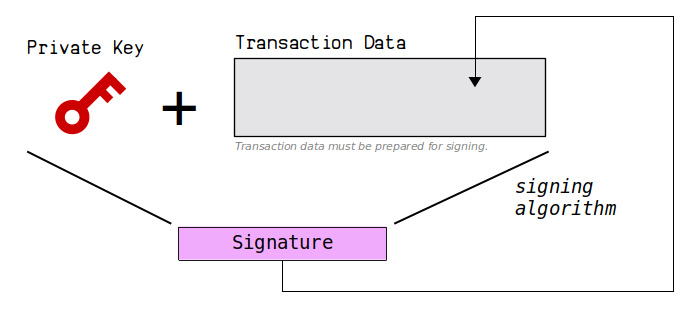When you want to send bitcoins to someone, you need to be able to provide a digital signature that prove you’ve got the `private key` for the `public key` that the bitcoins have been locked to.

This involves:

1. Constructing a transaction. This will be the message that we sign.
2. Using your private key to sign this message.
3. Putting this signature back inside the transaction.

Signing transactions in Bitcoin can be a little tricky, but it’s mostly just getting data in to the correct format. Here are the full steps for signing a classic bitcoin transaction:#### 0. Create a transaction.

To begin with, you want to create some transaction data that spends bitcoins that you own.

This is basically where you select the inputs you want to spend, and then create the outputs you want to lock those spent inputs to. In other words, it describes the movement of coins.I haven’t explained bitcoin transaction data structure here, so this part will be confusing if you’ve never seen a raw transaction before, but this is what one looks like:

``````Raw Transaction (Unsigned):

version: 01000000
inputs:  01
txid: b7994a0db2f373a29227e1d90da883c6ce1cb0dd2d6812e4558041ebbbcfa54b
vout: 00000000
scriptsigsize: 00
scriptsig:
sequence: ffffffff
outputs: 01
amount: 983a000000000000
scriptpubkeysize: 19
scriptpubkey: 76a914b3e2819b6262e0b1f19fc7229d75677f347c91ac88ac
locktime: 00000000
``````

The TXIDs used to reference inputs inside raw transaction data are actually in reverse byte order. This is a quirk of bitcoin.

Here I’ve selected the bitcoins I want to spend by referencing the `txid` and a specific `vout` (output) from a previous transaction, and I’ve created a new output containing an `amount` of bitcoins with a new `scriptpubkey` lock on it. This output uses a P2PKH lock, but that’s not important for now.

Most importantly, notice that the `scriptSig` for the input is currently empty. This is where an unlocking script containing our signature will go.

Anyway, we are now ready to sign this transaction data and “unlock” the input we’ve selected…

#### 1. Remove existing unlocking scripts

When we sign a transaction we only sign the data that describes the movement of coins. So if you’ve already created `scriptSig`s (unlocking scripts) for other inputs, remove them from the transaction temporarily.

In my transaction I’m only unlocking one input, so I don’t have to remove any existing `scriptSig`s.

#### 2. Put the locking script as a placeholder

Before signing an input, we need to put that input’s original locking script (`scriptpubkey`) in to the place where the signature for it is about to go (the `scriptsig`).

I’m not entirely sure why this is the case, but I’m guessing it helps to identify the specific input we want to sign, and also shows that we’re aware of the original locking script on the output we intend to spend.

Anyway, looking at the raw transaction data for this input we can see that it has a P2PKH locking script of `76a9144299ff317fcd12ef19047df66d72454691797bfc88ac`, so we put that in to the `scriptsig` as a placeholder:

``````Raw Transaction (Unsigned):

version: 01000000
inputs:  01
txid: b7994a0db2f373a29227e1d90da883c6ce1cb0dd2d6812e4558041ebbbcfa54b
vout: 00000000
scriptsigsize: 19
scriptsig: 76a9144299ff317fcd12ef19047df66d72454691797bfc88ac
sequence: ffffffff
outputs: 01
amount: 983a000000000000
scriptpubkeysize: 19
scriptpubkey: 76a914b3e2819b6262e0b1f19fc7229d75677f347c91ac88ac
locktime: 00000000
``````

Don’t forget that this placeholder will adjust the value of `scriptsigsize` temporarily too.

#### 3. Append signature hash type to transaction data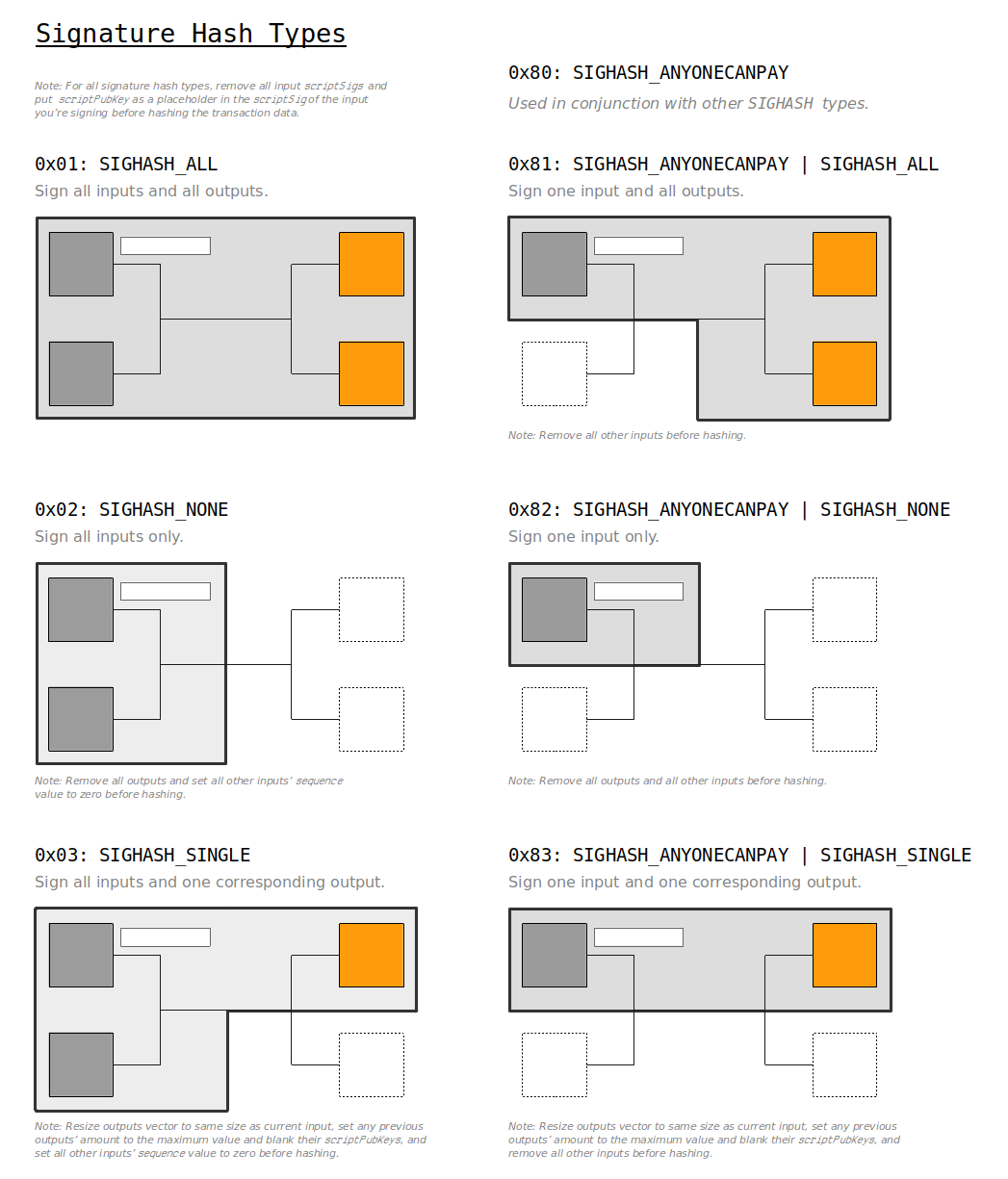``````Signature Hash Types:

0x01 = SIGHASH_ALL
0x02 = SIGHASH_NONE
0x03 = SIGHASH_SINGLE
0x81 = SIGHASH_ANYONECANPAY | SIGHASH_ALL
0x82 = SIGHASH_ANYONECANPAY | SIGHASH_NONE
0x83 = SIGHASH_ANYONECANPAY | SIGHASH_SINGLE
``````

At this point we can indicate how much of the transaction’s structure we are going to be signing by appending a signature hash type (`SIGHASH`) to the end of the transaction data.

The most common is `SIGHASH_ALL` (`0x01`), which indicates that the signature covers all of the inputs and outputs in the transaction, which means that nobody else can add any additional inputs or outputs to it later on.

So this is what our raw unsigned transaction looks like now:

``````Raw Transaction (Unsigned):

version: 01000000
inputs:  01
txid: b7994a0db2f373a29227e1d90da883c6ce1cb0dd2d6812e4558041ebbbcfa54b
vout: 00000000
scriptsigsize: 19
scriptsig: 76a9144299ff317fcd12ef19047df66d72454691797bfc88ac
sequence: ffffffff
outputs: 01
amount: 983a000000000000
scriptpubkeysize: 19
scriptpubkey: 76a914b3e2819b6262e0b1f19fc7229d75677f347c91ac88ac
locktime: 00000000
sighash: 01000000
``````

The `sighash` here is 4 bytes long, and also in little-endian byte order.

You may need to adjust the transaction data based on your choice of signature hash type. But for `SIGHASH_ALL` the current transaction structure is ready for signing.

#### 4. Hash the transaction data

Now that we’ve prepared the raw unsigned transaction, we can now create a hash of it ready for signing.

Bitcoin uses double SHA-256 when hashing things (also referred to hash “hash256”), so if we serialize our current unsigned transaction and hash it we get:

``````message: 0100000001b7994a0db2f373a29227e1d90da883c6ce1cb0dd2d6812e4558041ebbbcfa54b000000001976a9144299ff317fcd12ef19047df66d72454691797bfc88acffffffff01983a0000000000001976a914b3e2819b6262e0b1f19fc7229d75677f347c91ac88ac0000000001000000

``````

If we convert this hash to an integer we have:

``hash256(message): 75402077471587956851360588120356244127735644006942973877340910814730793844683``

`hash256(message)` is shorthand for `sha256(sha256(message))`. You almost always use double SHA-256 when hashing data in Bitcoin.

#### 5. Sign the transaction hash

Now we can just sign this message hash `z` like we would for any other message in ECDSA. All we need is our private key `d` and a randomly generated number `k`.

This is what the signature for this transaction looks like:

``````random number    (k): 123456789
hash256(message) (z): 75402077471587956851360588120356244127735644006942973877340910814730793844683
private key      (d): 112757557418114203588093402336452206775565751179231977388358956335153294300646

random point (k*G = R): {
x = 4051293998585674784991639592782214972820158391371785981004352359465450369227,
y = 88166831356626186178414913298033275054086243781277878360288998796587140930350
}

signature: r = R[x], s = k⁻¹ * (z + r * d): {
r = 4051293998585674784991639592782214972820158391371785981004352359465450369227,
s = 101656099268479774907861155236876278987061611115278341531512875302287938750185
}
``````

#### 6. Use the low-s value

Interestingly, there are actually two possible `s` values in ECDSA that will make for a valid signature. We call one the “high” `s` value and we call the other the “low” `s` value.

In mathematical terms, the “other” valid `s` value is just the additive inverse of our current `s` value in the finite field of `n`. As a result, both of these `s` values will actually get you to the same x-coordinate of the random point `R` when doing signature verification.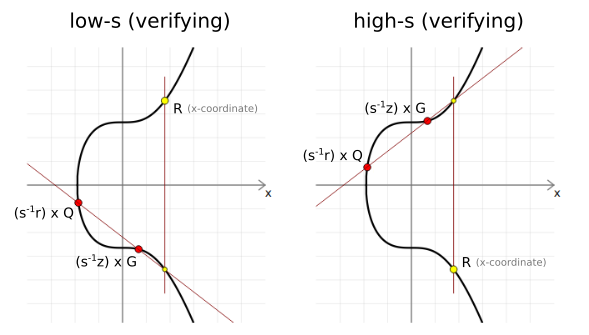The additive inverse of s value calculates the opposite points on the curve during signature validation, but the x-coordinate of the third point will be the same.

Anyway, in Bitcoin this feature is a bit annoying, because it means that anyone could invert the `s` value in our signature after we send our transaction in to the network, and this would alter the resulting TXID. This doesn’t change the actual structure of the transaction (the money moves to the same place in the same way), but it does mean we lose the ability to reliably keep track of the transaction in the blockchain.

So to help remedy this high/low `s` value problem, BIP 62 introduced a rule where all signatures should use the low `s` value by default, otherwise the transaction is considered non-standard2 and will not be relayed by nodes.

You’ll know if you’ve ended up with the “high” `s` value because it will be in the upper half of the finite field of `n` (i.e. `s > n/2`). If this is the case (such as with our `s` value), we just subtract `s` value from `n` to give us the “low” `s` value:

``````n     = 115792089237316195423570985008687907852837564279074904382605163141518161494337

s     = 101656099268479774907861155236876278987061611115278341531512875302287938750185  <- high s value
n - s = 14135989968836420515709829771811628865775953163796562851092287839230222744152   <- low s value
``````

In Ruby code this is as simple as:

``````if (signature[:s] > \$n / 2)
signature[:s] = \$n - signature[:s]
end``````

As I say, both `s` values are technically valid – it’s just in Bitcoin we use the low `s` value to help prevent transaction malleability.

#### 7. DER encode the signature

The next step is to format the signature ready to be put inside a bitcoin transaction.

Bitcoin uses DER encoding for signatures, which is a bit verbose, but it’s what Satoshi chose, so now we have to use it when encoding signatures for transactions.

Why did Satoshi decided to use DER encoding?…

My guess is that Satoshi did not know about the internals of ECDSA signatures, and simply used what OpenSSL gave him. If it didn’t require a hard forking change (requiring every wallet and verifying node on the network to upgrade), we’d have changed it long ago.

Pieter Wuille

Anyway, DER encoding signatures in Bitcoin basically involves converting the `r` and `s` values to hexadecimal, and adding a few bytes in between to indicate the length and type of data. There’s also a quirk where if the first byte of `r` or `s` is `0x80` or above, we prepend a zero `0x00` byte to them respectively. But other than that, it’s pretty straightforward.

So if this is our raw signature:

``````signature: {
r = 4051293998585674784991639592782214972820158391371785981004352359465450369227,
s = 14135989968836420515709829771811628865775953163796562851092287839230222744152
}
``````

This is what it looks like in DER encoding:

``````der encoded:
type: 30
length: 44
type:   02
length: 20
r:      08f4f37e2d8f74e18c1b8fde2374d5f28402fb8ab7fd1cc5b786aa40851a70cb
type:   02
length: 20
s:      1f40afd1627798ee8529095ca4b205498032315240ac322c9d8ff0f205a93a58
``````

The type bytes `0x30` and `0x20` are always the same. It’s only the length and actual data fields that you need to change when encoding a signature in DER format.

See this stackexchange.com post for more details.

#### 8. Append signature hash type to DER encoded signature

After encoding our signature in to DER format we once again append the `SIGHASH` type to indicate how much of the transaction data this signature applies to. This must be the same as the signature hash type we chose in step 3.

So this is what our encoded signature looks like now:

``````der encoded (with SIGHASH):
type: 30
length: 44
type:   02
length: 20
r:      08f4f37e2d8f74e18c1b8fde2374d5f28402fb8ab7fd1cc5b786aa40851a70cb
type:   02
length: 20
s:      1f40afd1627798ee8529095ca4b205498032315240ac322c9d8ff0f205a93a58
sighash: 01
``````

The `SIGHASH` type here is 1 byte, even though the `SIGHASH` type we appended to the transaction data before hashing is 4 bytes. This is just another quirk of bitcoin.3

The reason we appended the `SIGHASH` type before hashing is because it allows us to commit to that `SIGHASH` type before we create the signature. In other words, if someone changes this second value to something like `SIGHASH_ANYONECANPAY` (which suggests anyone could add more inputs to the transaction), it will not match the `SIGHASH` value we chose when we hashed and signed the initial transaction data.

Anyway, if we serialize all of this data our encoded signature looks like:

``````der encoded (with SIGHASH):
3044022008f4f37e2d8f74e18c1b8fde2374d5f28402fb8ab7fd1cc5b786aa40851a70cb02201f40afd1627798ee8529095ca4b205498032315240ac322c9d8ff0f205a93a5801
``````

#### 9. Construct unlocking script

As a final step, we need to place this signature in to a script that will unlock the the input.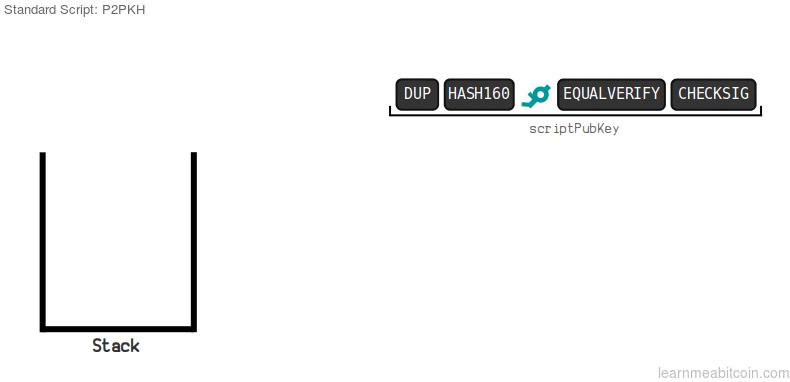I’m not going to cover the mechanics of script here, but let’s just say that our chosen input has the following P2PKH locking script (`scriptpubkey`) on it:

``````scriptpubkey:

asm: OP_DUP OP_HASH [length] [public key hash] OP_EQUALVERIFY OP_CHECKSIG
hex: 76a9144299ff317fcd12ef19047df66d72454691797bfc88ac
``````

In short, this input has been locked to the hash of our public key. To unlock it, we need to provide a valid `signature` alongside the original `public key`.

So if this is the `public key` and our `signature`:

``````public key: 024aeaf55040fa16de37303d13ca1dde85f4ca9baa36e2963a27a1c0c1165fe2b1
signature:  3044022008f4f37e2d8f74e18c1b8fde2374d5f28402fb8ab7fd1cc5b786aa40851a70cb02201f40afd1627798ee8529095ca4b205498032315240ac322c9d8ff0f205a93a5801
``````

This is what our unlocking script (`scriptsig`) looks like:

``````scriptpubkey:

asm: [signature length] [signature] [public key length] [public key]
hex: 473044022008f4f37e2d8f74e18c1b8fde2374d5f28402fb8ab7fd1cc5b786aa40851a70cb02201f40afd1627798ee8529095ca4b205498032315240ac322c9d8ff0f205a93a580121024aeaf55040fa16de37303d13ca1dde85f4ca9baa36e2963a27a1c0c1165fe2b1
``````

This is just the DER-encoded signature followed by the public key, with `0x47` and `0x21` bytes before each to indicate their length.

#### 10. Insert the unlocking script in to the transaction

Finally, we just pop this unlocking script in to the transaction:

``````Raw Transaction (Signed):

version: 01000000
inputs:  01
txid: b7994a0db2f373a29227e1d90da883c6ce1cb0dd2d6812e4558041ebbbcfa54b
vout: 00000000
scriptsigsize: 6a
scriptsig: 473044022008f4f37e2d8f74e18c1b8fde2374d5f28402fb8ab7fd1cc5b786aa40851a70cb02201f40afd1627798ee8529095ca4b205498032315240ac322c9d8ff0f205a93a580121024aeaf55040fa16de37303d13ca1dde85f4ca9baa36e2963a27a1c0c1165fe2b1
sequence: ffffffff
outputs: 01
amount: 983a000000000000
scriptpubkeysize: 19
scriptpubkey: 76a914b3e2819b6262e0b1f19fc7229d75677f347c91ac88ac
locktime: 00000000
``````

Note: Repeat steps 1-9 for each input you want to unlock.

Finally, if we serialize this transaction data we get:

``````Raw Transaction (Signed):

0100000001b7994a0db2f373a29227e1d90da883c6ce1cb0dd2d6812e4558041ebbbcfa54b000000006a473044022008f4f37e2d8f74e18c1b8fde2374d5f28402fb8ab7fd1cc5b786aa40851a70cb02201f40afd1627798ee8529095ca4b205498032315240ac322c9d8ff0f205a93a580121024aeaf55040fa16de37303d13ca1dde85f4ca9baa36e2963a27a1c0c1165fe2b1ffffffff01983a0000000000001976a914b3e2819b6262e0b1f19fc7229d75677f347c91ac88ac00000000
``````

And that’s a valid transaction that we can send in to the bitcoin network.

#### `bitcoin-cli sendrawtransaction`

Send some raw transaction data in to the bitcoin network. For example:

``\$ bitcoin-cli sendrawtransaction 0100000001b7994a0db2f373a29227e1d90da883c6ce1cb0dd2d6812e4558041ebbbcfa54b000000006a473044022008f4f37e2d8f74e18c1b8fde2374d5f28402fb8ab7fd1cc5b786aa40851a70cb02201f40afd1627798ee8529095ca4b205498032315240ac322c9d8ff0f205a93a580121024aeaf55040fa16de37303d13ca1dde85f4ca9baa36e2963a27a1c0c1165fe2b1ffffffff01983a0000000000001976a914b3e2819b6262e0b1f19fc7229d75677f347c91ac88ac00000000``

The following code shows how you can sign a transaction using the steps above:

``````# A basic structure for holding the transaction data
def tx(scriptsig)
# Need to calculate a byte indicating the size of upcoming scriptsig in bytes (rough code but does the job)
size = (scriptsig.length / 2).to_s(16).rjust(2, "0")

# Raw unsigned transaction data with the scriptsig field (you need to know the correct position)
return "0100000001b7994a0db2f373a29227e1d90da883c6ce1cb0dd2d6812e4558041ebbbcfa54b00000000#{size}#{scriptsig}ffffffff01983a0000000000001976a914b3e2819b6262e0b1f19fc7229d75677f347c91ac88ac00000000"
end

# Private key and public key for the locked up bitcoins we want to spend
private_key = "f94a840f1e1a901843a75dd07ffcc5c84478dc4f987797474c9393ac53ab55e6" # sha256("learnmeabitcoin1")
public_key = "024aeaf55040fa16de37303d13ca1dde85f4ca9baa36e2963a27a1c0c1165fe2b1"

# NOTE: Need to remove all existing signatures from the transaction data first if there are any

# Put original scriptpubkey as a placeholder in to the scriptsig for the input you want to sign (required)
scriptpubkey = "76a9144299ff317fcd12ef19047df66d72454691797bfc88ac" # just one input in this transaction
transaction = tx(scriptpubkey)

# Append sighash type to transaction data (required)
transaction = transaction + "01000000"

# Get a hash of the transaction data (because we sign the hash of data and not the actual data itself)
hash = Digest::SHA256.hexdigest(Digest::SHA256.digest([transaction].pack("H*")))

# Use elliptic curve mathematics to sign the hash using the private key and nonce
signature = sign(private_key.to_i(16), hash.to_i(16), 123456789) # using a fixed nonce for testing (unsafe)

# Use the low s value (BIP 62: Dealing with malleability)
if (signature[:s] > \$n / 2)
signature[:s] = \$n - signature[:s]
end

# Encode the signature in to DER format (slightly awkward format used for signatures in bitcoin transactions)
r = signature[:r].to_s(16).rjust(64, "0")  # convert r to hexadecimal
s = signature[:s].to_s(16).rjust(64, "0")  # convert s to hexadecimal
r = "00" + r if (r[0, 2].to_i(16) >= 0x80) # prepend 00 if first byte is 0x80 or above (DER quirk)
s = "00" + r if (s[0, 2].to_i(16) >= 0x80) # prepend 00 if first byte is 0x80 or above (DER quirk)
der = ""                                   # string for holding our der encoding
r_len = (r.length / 2).to_s(16).rjust(2, "0") # get length of r (in bytes)

s_len = (s.length / 2).to_s(16).rjust(2, "0") # get length of s (in bytes)
der << "02" << r_len << r << "02" << s_len << s   # Add to DER encoding (0x20 byte indicates an integer type in DER)
der_len = (der.length / 2).to_s(16).rjust(2, "0") # get length of DER data (in bytes)
der = "30" + der_len + der # Final DER encoding (0x30 byte incatetes compound object type)

# Append sighashtype to the signature (required) (01 = ALL)
der = der + "01" # without it you get "mandatory-script-verify-flag-failed (Non-canonical DER signature) (code 16)"

# Contruct full unlocking script (P2PKH scripts need original public key the bitcoins were locked to): <size> {signature} <size> {public_key}
scriptsig = (der.length / 2).to_s(16) + der + (public_key.length / 2).to_s(16) + public_key

# Put the full scriptsig in to the original transaction data
transaction = tx(scriptsig)

# Show the signed transaction
puts transaction #=> 0100000001b7994a0db2f373a29227e1d90da883c6ce1cb0dd2d6812e4558041ebbbcfa54b000000006a473044022008f4f37e2d8f74e18c1b8fde2374d5f28402fb8ab7fd1cc5b786aa40851a70cb02201f40afd1627798ee8529095ca4b205498032315240ac322c9d8ff0f205a93a580121024aeaf55040fa16de37303d13ca1dde85f4ca9baa36e2963a27a1c0c1165fe2b1ffffffff01983a0000000000001976a914b3e2819b6262e0b1f19fc7229d75677f347c91ac88ac00000000

# Send the transaction in to the bitcoin network
# \$ bitcoin-cli sendrawtransaction [raw transaction data]``````

It can be quite tricky preparing the data for signing, so it may take a few attempts to get it right. The DER encoding looks especially complicated, but ultimately it’s just the `r` and `s` values of the signature with a few bytes thrown in between to indicate the length of data.

## Summary

The best way to get the hang of ECDSA is to try coding it yourself.

The hardest part is not usually the elliptic curve mathematics, but preparing and formatting the signatures for use inside bitcoin transactions. Furthermore, not all programming languages make it easy to work with big numbers, so you may need to use special functions to perform the elliptic curve operations.

Other than that, the code is not as difficult as you may have initially thought.

Of course, I wouldn’t recommend using this code in your most recent mission-critical system, but it should help you get started with creating your own public keys and signing your own transactions in Bitcoin.

Have fun.

References

• NIST.FIPS.186-4.pdf - Official Digital Signature Standard by NIST. Contains outlines for DSA, RSA, and ECDSA.
• sec2-v2.pdf - List of recommended curves for use in elliptic curve cryptography from SECG. Contains parameters for the `secp256k1` curve used in Bitcoin.

Visualisations

• Elliptic Curve Plotter - A small but cool program that allows you to play with simple elliptic curve operations. It’s what I used to help create the diagrams on this page.
• Sage Math - A big mathematical library that comes with elliptic curve plotting functions. I used it to show the plots of elliptic curves over real numbers and over finite fields.
• Interactive Elliptic Curve Operations - A cool web tool created by Andrea Corbellini that allows you to visualise elliptic curve addition and double operations over both real numbers and a finite field.

Explanations

Code

Here are some implementations of ECDSA in different programming languages that I found helpful:

Other

I must admit, this project was 2 years of development before release, and I could only spend so much time on each of the many issues. I found guidance on the recommended size for SHA and RSA, but nothing on ECDSA which was relatively new. I took the recommended key size for RSA and converted to equivalent key size for ECDSA, but then increased it so the whole app could be said to be 256-bit security. I didn’t find anything to recommend a curve type so I just… picked one. Hopefully there is enough key size to make up for any deficiency.

Satoshi Nakamoto

Hey there, it's Greg.

I'll let you know about cool website updates, or if something seriously interesting happens in bitcoin.

Don't worry, it doesn't happen very often.